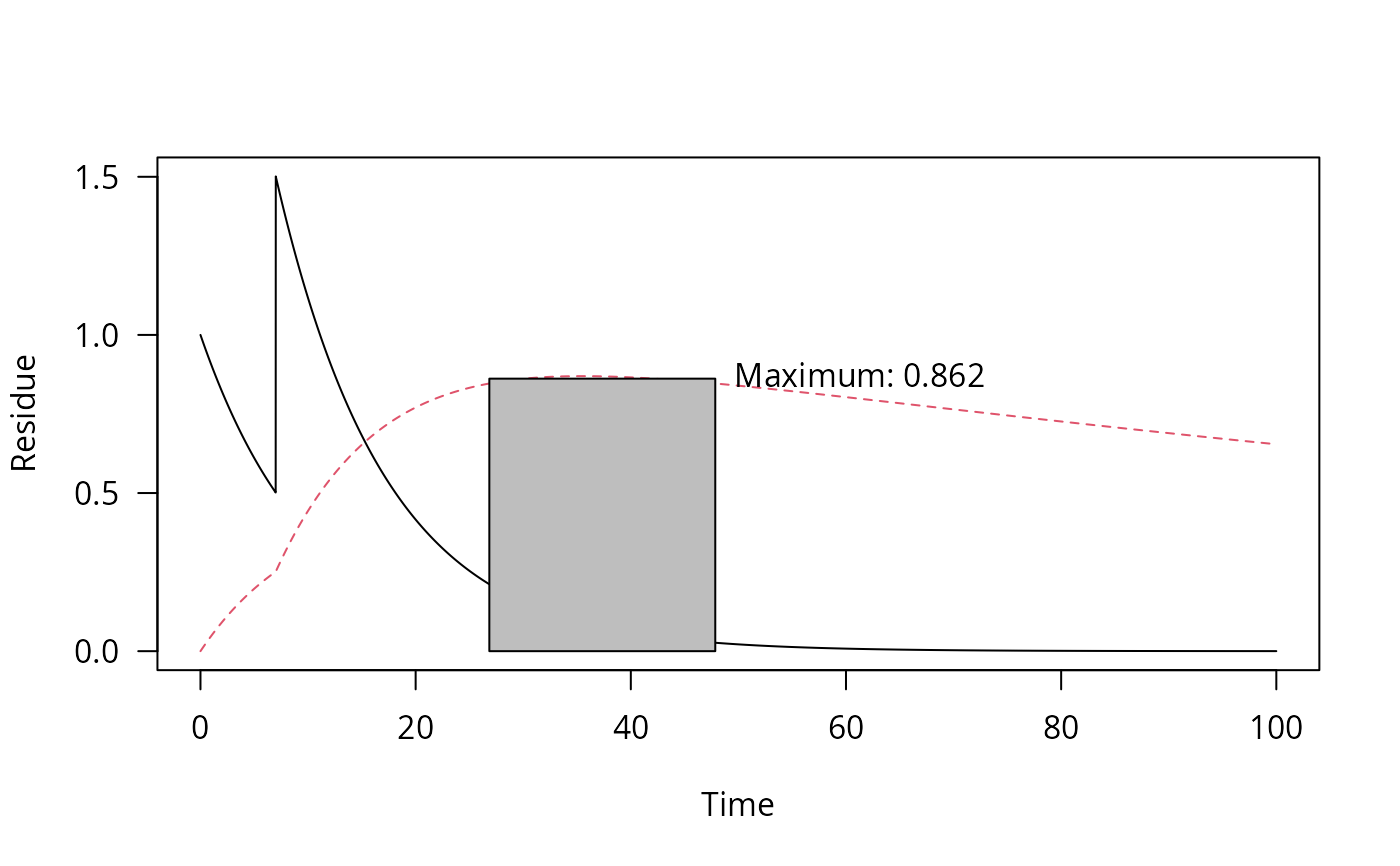If the application pattern is specified in applications, n and i are disregarded.

sawtooth(
x,
n = 1,
i = 365,
applications = data.frame(time = seq(0, (n - 1) * i, length.out = n), amount = 1)
)

## Arguments

x A one_box object The number of applications. If applications is specified, n is ignored The interval between applications. If applications is specified, i is ignored A data frame holding the application times in the first column and the corresponding amounts applied in the second column.

## Examples

applications = data.frame(time = seq(0, 14, by = 7), amount = c(1, 2, 3))
pred <- one_box(10)
plot(sawtooth(pred, applications = applications))m_2 <- mkinmod(parent = mkinsub("SFO", "m1"), m1 = mkinsub("SFO"))#> Successfully compiled differential equation model from auto-generated C code.fit_2 <- mkinfit(m_2, FOCUS_2006_D, quiet = TRUE)#> Warning: Observations with value of zero were removed from the data#> Warning: Shapiro-Wilk test for standardized residuals: p =  0.0165pred_2 <- one_box(fit_2, ini = 1)
pred_2_saw <- sawtooth(pred_2, 2, 7)
plot(pred_2_saw, max_twa = 21, max_twa_var = "m1")max_twa(pred_2_saw)#> $max #> parent m1 #> 0.7834480 0.8617048 #> #>$window_start
#> parent     m1
#>   0.00  26.85
#>
#> \$window_end
#> parent     m1
#>  21.00  47.85
#>